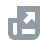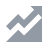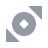Markets
Futures
Earn
More
Cancel
FAQAccount FunctionsTutorialBinance Fan TokenBinance EarnCrypto Deposit/WithdrawalCrypto DerivativesFinanceAPISecurityBinance ConvertNFTVIP
Home
Support Center
FAQ
Crypto Derivatives
Futures Contracts
Introduction to Binance Futures
How to Calculate Profit and Loss for Futures Contracts

# How to Calculate Profit and Loss for Futures Contracts

2021-07-28 07:06
Profit and loss calculations are based on the contract’s collateral. For example, a USDⓈ-margined contract is denominated in USDT, whereas a BTC COIN-margined contract is denominated in BTC.
Please note that unrealized profit and loss are calculated based on the Mark Price, while realized profit and loss are calculated based on the Last Price.

## Profit and Loss calculations for COIN-M Futures contracts (BTCUSD)

A BTC COIN-M Futures contract is denominated, collateralized, and settled in Bitcoin, which means Bitcoin is used as the base currency. Each BTC COIN-M contract represents 100 USD and as such, USD is the counter currency. Since each contract represents a fixed quantity of USD, this means BTC is used to fund the Initial Margin or calculate profit and loss.
Suppose you purchased 100 BTC-margined perpetual contracts (100 * \$100 = \$10,000) at \$50,000 each. By doing this, you are essentially selling \$10,000 and buying an equivalent value of Bitcoin (10,000 / 50,000 = 0.2 BTC).
Suppose Bitcoin’s price rose to \$55,000 and you want to secure profits from the trade. To close the position, you buy back \$10,000 worth of contracts and simultaneously sell the equivalent in Bitcoin (10,000 / 55,000 = 0.1818 BTC).
In this trade, your profit will be calculated as: Quantity of Bitcoins at Entry - Quantity of Bitcoins at Exit = 0.2 - 0.1818 = 0.0182 BTC.
In short, the profit and loss formula will be:
((1 / Futures Entry Price) - (1 / Futures Exit Price)) * Position Size
((1/ 50,000) - (1 / 55,000)) * (100 * 100) = 0.0182 BTC
Example for short position:
Short BTCUSD 0925 Quarterly (Buy USD, Sell BTC):
((1 / Futures Entry Price) - (1 / Futures Exit Price)) * (Position Size * -1)
((1/50,000) - (1/45,500)) * (100 contracts * 100 USD * -1) = 0.0198 BTC

## Profit and Loss calculations for USDⓈ-M Futures contracts (BTCUSDT)

Suppose you purchase (long) 10,000 USDT worth of BTCUSDT perpetual contracts at 50,000 USDT. The price increases and you exit at 55,000 USDT. Your profit will be:
((1 / Futures Entry Price) - (1 / Futures Exit Price)) * Position Size
( 1 / 50,000 - 1 / 55,000 ) * 10,000 = 0.018182 BTC
In USDT, it would be 0.018182 * 55,000 USDT = 1,000 USDT
Suppose you sold (short) 10,000 USDT worth of BTCUSDT perpetual contracts at 50,000 USDT. The price decreases and you exit at 45,000 USDT. Your profit will be:
((1 / Futures Entry Price) - (1 / Futures Exit Price)) * (Position Size * -1)
( 1 / 50,000 - 1 / 45,000 ) * -10,000 = 0.022 BTC
In USDT, it would be 0.022 * 45,000 USDT = 1,000 USDT

## How to calculate Unrealized PNL and ROE percentage?

You can use the Mark Price or the Entry Price to calculate the Return on Equity (ROE). Go to the [Positions] tab on the trading interface, click [PnL(ROE%)] and select the desired price basis.

### USDⓈ-M Futures Contracts

• If you chose the Mark Price as the price basis:
Unrealized PNL = Position Size * Direction of Order * (Mark Price - Entry Price)
ROE% = Unrealized PNL in USDT / Entry Margin = ( (Mark Price - Entry Price ) * Direction of Order * Size ) / (position_amount * contract_multiplier * mark_price * IMR)
*IMR = 1 / Leverage
• If you chose the Last Price as the price basis:
Unrealized PNL = Position Size * Direction of Order * (Last Price - Entry Price)
ROE% = Unrealized PNL in USDT / Entry Margin = ( (Last Price - Entry Price) * Direction of Order * Size) / (position_amount * contract_multiplier * mark_price * IMR)
Direction of order: 1 for long orders; -1 for short orders

### COIN-M Futures Contracts

Unrealized PNL = position_size * contract_multiplier * Direction of Order * (1 / Entry Price - 1 / Mark Price)
ROE% = Unrealized PNL * price / abs(size) * contract_multiplier * IMR
Please note that "price" in the above formula could be the Mark Price or the Entry Price (based on the price basis).